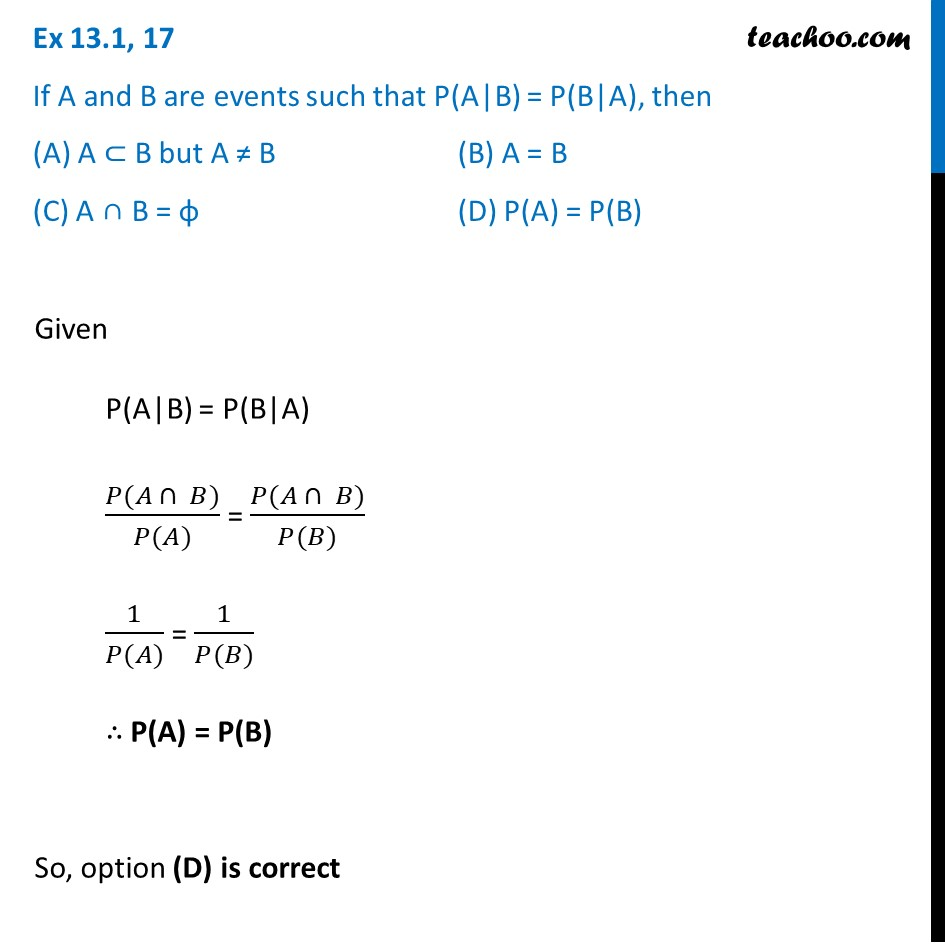Ex 13.1

Chapter 13 Class 12 Probability
Serial order wiseLearn in your speed, with individual attention - Teachoo Maths 1-on-1 Class

### Transcript

Ex 13.1, 17 If A and B are events such that P(A|B) = P(B|A), then (A) A ⊂ B but A ≠ B (B) A = B (C) A ∩ B = φ (D) P(A) = P(B)Given P(A|B) = P(B|A) (𝑃(𝐴 ∩ 𝐵))/(𝑃(𝐴)) = (𝑃(𝐴 ∩ 𝐵))/(𝑃(𝐵)) 1/(𝑃(𝐴)) = 1/(𝑃(𝐵)) ∴ "P(A) = P(B)" So, option (D) is correct